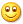maik9898 赛菲特

# 可公度性在股市中的运用

[2012-05-01 11:26:27]

“可公度性”一词是在天文学中首先提出来的。由于至今还没有人能够提出有说服力的机制理论，所以一直当作经验关系写入某些文献中。笔者认为可公度性的实质是——利用数学模式反映出事物本身或相关事物之间的运动关系中隐含的周期性规律，它是周期性规律的扩张化应用，在增加错误（或虚惊）概率的代价下，可以从很宽的范围内分辨并抽提出微弱的、非偶然的信号，类似于遗传算法中的自我隔代复制。例如大家都知道上证指数的一个主要低点循环周期是18-20个月，除此之外还隐藏哪些周期，一时半会还不容易发现，而且当大部分人都在利用这个数据时，它的有效性就大打折扣，按以前的规律2002年10月应该是循环低点，实际上去年10月只出现了一次反弹。可公度性就能很好地解决这个问题。
拉普拉斯在200多年前注意到木星的三个主要卫星的平均运动Z1，Z2，Z3服从下列关系式：Z1-3×Z2+2×Z3=0。同样，土星的四个卫星的平均运动Y1，Y2，Y3，Y4也具有类似的关系：5×Y1-10×Y2+Y3+4×Y4=0。我们就称这些卫星之间存在可公度性。

我们利用可公度性对上证指数进行中远期重要转折点预测，得到非常好的效果，以下为预测方法。
首先解决选点问题，根据混沌理论中的分形理论原理，底部转折点的前一点和后一点均比转折点高，顶部转折点的前一点和后一点均比转折点低。其次，起点取上证指数历史上第一个重要的转折点。另由于上证指数第一个交易日为1990年12月19日，所以月份的划分以每月18日为界。
以下为1991年5月18日上证指数104点以来历次主要低点的时间跨度表， 计算方式如下：
设1991年5月18日为0点，低点时间在当月18日之前的计算方法为
时间跨度=（年份-1991）*12-5+月份
低点时间在当月18日之后的只要再加上1即可。
序号    指数时间（点位）    时间跨度        序号    指数时间（点位）    时间跨度
X0    1991.05.17（104）    0        X11    1998.08.18（1043）    87
X1    1992.11.17（386）    18        X12    1999.02.08（1064）    93
X2    1993.03.25（913）    23        X13    1999.05.17（1047）    96
X3    1993.07.27（777）    27        X14    1999.12.27（1341）    104
X4    1993.10.25（774）    30        X15    2000.09.25（1874）    113
X5    1994.07.29（325）    39        X16    2001.02.22（1893）    118
X6    1995.02.07（524）    45        X17    2001.10.22（1514）    126
X7    1995.07.04（610）    50        X18    2002.01.29（1339）    129
X8    1996.01.19（512）    56        X19    2002.06.06（1455）    133
X9    1996.12.25（855）    68        X20    2003.01.06（1311）    140
X10    1997.09.23（1025）    77
我们看到X20以前数据基本上可以找到可公度性的规律，比如：
X20的低点可以根据以下二元公式数据组得出：X2+X16=23+118=141，
X3+X15=27+113=140，X6+X13=45+96=141，
X19的低点可以根据以下二元公式数据组得出：X4+X14=30+104=134，X5+X12=39+93=132，X6+X11=45+87=132，X8+X10=56+77=133
其他数据也可以根据类似的方法找出，
根据以上低点数据目前我们采用二元公式，即把数字两两相加，得出如下数据组：
X4+X15=30+113=143，X5+X14=39+104=143，X8+X11=56+87=143，X1+X17=18+126=144
143指向今年3月，144指向今年4月，也就是说今年3—4月有可能出现一个低点或者次低点。
另外我们可以看到另一组数据：
X1+X19=18+133=151，X2+X18=23+129=152，X5+X15=39+113=152，X8+X13=56+96=152，
152指向今年12月附近，也就是说今年底有可能出现另一个低点。意味着我们在年底之前要选择高点时间区做一次全面的减仓出货。
天文学的可公度性在股市中的运用（2）
——高点的可公度性
上次我们对低点的可公度性作了一定的分析，那么它能否用在高点的数据中呢？
下面让我们看看效果如何。
计算方式如下：
设1992年5月24日为0点，高点时间在当月18日之前的计算方法为
时间跨度=（年份-1992）*12-5+月份-1
高点时间在当月18日之后的只要不减1即可。
序号    指数时间（点位）    时间跨度        序号    指数时间（点位）    时间跨度
X0    1992.05.24（1429）    0        X11    1997.09.11（1264）    63
X1    1993.02.16（1558）    8        X12    1998.06.04（1422）    72
X2    1993.04.29（1392）    11        X13    1998.11.17（1300）    77
X3    1993.08.17（1042）    15        X14    1999.06.30（1756）    85
X4    1993.12.08（1044）    18        X15    1999.09.10（1695）    87
X5    1994.09.13（1052）    27        X16    2000.08.22（2114）    99
X6    1995.05.22（927）    36        X17    2001.01.08（2131）    103
X7    1995.09.12（790）    39        X18    2001.06.14（2245）    108
X8    1996.07.24（894）    50        X19    2001.12.05（1776）    114
X9    1996.12.11（1258）    54        X20    2002.03.21（1693）    118
X10    1997.05.12（1510）    59        X21    2002.06.25(1748)    121
首先，我们可以看到以前的数据基本上可以找到可公度性的规律，比如：
X21的高点可以根据以下二元公式数据组得出：X1+X19=8+114=122，X4+X17=18+103=121，
X6+X14=36+85=121，X8+X12=50+72=122，X10+X11=59+63=122
有这么多的时间周期汇集，难怪去年的六、七月份是铁顶，
X20的高点可以根据以下二元公式数据组得出：X2+X18=11+108=119，X3+X17=15+103=118
X4+X16=18+99=117，X9+X11=54+63=117，X10+X10=59+59=118
其次根据以上高点数据我们采用二元公式，即把数字两两相加，得出如下数据组：
X1+X21=8+121=129，X2+X20=11+118=129，X3+X19=15+114=129，X5+X17=27+103=130，
129指向今年2月附近，所以今年2月在高位反复震荡都无法向上突破。
今年下一个重要高点数据指向哪呢？看看以下数据组：
X1+X21=11+121=132，X3+X20=15+118=133，X4+X19=18+114=132，X9+X13=54+77=131，
X10+X12=59+72=131，
131指向今年4月，132指向今年5月，也就是说今年4—5月有可能出现一个高点。
另一个数据组：
X4+X20=18+118=136，X5+X18=27+108=135，X6+X16=36+99=135，X8+X14=50+85=135，
X10+X13=59+77=136，X11+X12=63+72=135
135指向今年8月，136指向今年9月，也就是说今年8—9月有可能出现一个重要高点或者次高点。如果在那时还有股票的朋友需要特别小心了。结合上次预测的低点信号，我们认为第四季度股票持仓率应相对保守。
天文学的可公度性在股市中的运用（3）
——拓展可公度性原理及其误差纠正
根据可公度性的原理，按照公历日计算，以1990年12月为起点0，平均每隔9个月左右就出现一个重要转折点。如下表
时间跨度     年份月份    与转折点的误差        时间跨度     年份月份    与转折点的误差
0    1990.12    上交所开市        81    1997.9    高点
9    1991.9    无转折点        90    1998.6    高点
18    1992.6    （-1）为高点        99    1999.3    （+1）为高点
27    1993.3    （-1）为高点        108    1999.12    低点
36    1993.12    高点        117    2000.9    （-1）为高点
45    1994.9    高点        126    2001.6    高点
54    1995.6    （-1）为高点        135    2002.3    高点
63    1996.3    低点        144    2002.12    （+1）为低点
72    1996.12    高点        153    2003.9
这些转折点几乎都是高点，同样可以推断出今年9月份左右成为高点或者次高点的概率很高。
任何预测都会存在误差，可公度性同样也不例外，如何回避错误、减少误差，使它成为严谨的、科学的、稳定的、有效的预测方法，笔者总结出以下几点：
1、    必须注意数据的分布密度，分布密度有两重含义1》同系列数据内部的比较，象去年高点中六、七月份的密度明显比三月份的高，前者数据为2个121、3个122，后者数据为2个117、2个118、1个119，虽然两者都是五组数据，但前者只与两个数有关，所以更重要一些，六、七月份之后基本上就没有大的反弹。2》不同系列（高、低点）数据之间的比较，象去年12月份的高点数据组非常多，包括X1+X20=126，X2+X19=125，X4+X18=126，X5+X16=126，X7+X15=126，X8+X13=127，X9+X12=126，X11+X11=126，低点数据组偏少。所以，虽然指数已经大幅下跌了不少，但是反弹后仍然继续创新低。
2、    必须充分运用反证，高、低点数据有时会有冲突，出现重叠。这时无论是高点还是低点的有效性都大打折扣，行情一般会出现大的波动，并不是重要的转折点，如果高、低点的数据没有冲突时就要特别关注。

500/500